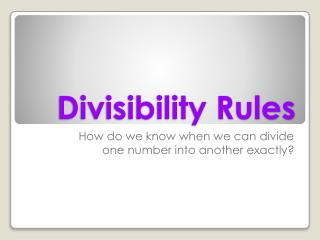# Divisibility Rules - PowerPoint PPT PresentationDownload PresentationDivisibility Rules

Divisibility Rules
Download Presentation## Divisibility Rules

- - - - - - - - - - - - - - - - - - - - - - - - - - - E N D - - - - - - - - - - - - - - - - - - - - - - - - - - -
##### Presentation Transcript

1. Divisibility Rules How do we know when we can divide one number into another exactly?

2. Math Challenge Create a five digit number that is divisible by 2, 5, and 10. Create a five digit number that is divisible by 2, but not 5 or 10. Can you create a number that is divisible by 5, but not by ten?

3. Today we will study the Rules of DivisibilityHow do you know if a number is divisible by 2? What about 3? 4? 5? Discuss what you know about divisibility with your partner or group.

4. Divisibility Rules for 2 A number is divisible by 2 if... • the last digit is even

5. Divisibility Rules for 3 A number is divisible by 3 if... • the sum of the digits is 3, 6 or 9

6. Divisibility Rules for 4 A number is divisible by 4 if... • the number made by the last two digits can be divided by 4

7. Divisibility Rules for 5 A number is divisible by 5 if • the last digit is a 5 or a 0

8. Divisibility Rules for 6 A number can be divided by 6 if • the last digit is even and the sum of all the digits is 3, 6 or 9

9. Divisibility Rules for 8 A number is divisible by 8 if • the number made by the last three digits will be divisible by 8

10. Divisibility Rules for 9 A number is divisible by 9 if • the sum of all the digits will add to 9

11. Divisibility Rules for 10 A number is divisible by 10 if • the last digit is a 0

12. Divisibility Rules for 7 Can you create a divisibility rule for the number 7?